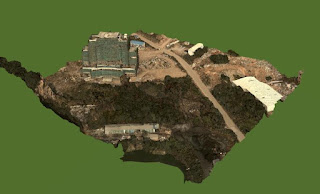# 3DTiles:glTF with RCT extension for NEU coordinate system

I try to convert osgb into 3d tiles and I think that glTF with RTC extension does not support NEU coordinate system. Am I right?

In my understanding, for most photogrammetry data, it is NEU(D) coordinate system with geometry positions(deltaPos array), therefore, when we render it in SCENE3D mode,we just get the Matrix from NEU to WGS84(1) and calculate the positionwc(2) as follows:

Matrix(Centre) = M(Rotation) * M(Translation);(1)

positionwc = Matrix(Centre) * deltaPos;(2)

But it seems that glTF only supports plane scene mode(Axis::Y_UP), so CESIUM_RTC_MODELVIEW only considers M(Translation) but ignores M(Rotation), so when I generate 3d tiles data, I have to correct all positions following this formula:

positionwc = Matrix(Centre) * deltaPos = M(Rotation) * M(Translation) * deltaPos = M(Translation) * deltaPos(glTF);(3)

deltaPos(glTF) = M(Translation).Inverse() * M(Rotation) * M(Translation) * deltaPos;(4)

I personally think it could be better to avoid this redundant calculation process to get the deltaPos(glTF) array, so we just copy the original position data into glTF data buffer; then we add NEU mode in the Cesium::Axis and gltfUpAxis; finally, we only need to modify several codes in Cesium: the first is the function Model::updateNodeHierarchyModelMatrix(), and the second is the uniform CESIUM_RTC_MODELVIEW.

The attachment pictures are my simple modification in Cesium. I tested it and it is practical. The benefits are these: 1, reducing the calculation during generating 3dtiles data, improving the performance; 2, supporting to show it on any positions in different mode（3D, 2.5D, 2D） around the earth 3 just several simple modifications in Cesium::Model

//in updateNodeHierarchyModelMatrix() function, recalculate the boundingsphere

if (defined(model._rtcCenter)) {

heading = pitch = roll = 0.0;

var mvRtc = new Matrix4();

var v4 = new Cartesian4(command.boundingVolume.center.x,command.boundingVolume.center.y,command.boundingVolume.center.z,1);

Matrix4.multiplyByVector(mvRtc,v4,v4);

command.boundingVolume.center.x = v4.x;

command.boundingVolume.center.y = v4.y;

command.boundingVolume.center.z = v4.z;

}

CESIUM_RTC_MODELVIEW : function(uniformState, model) {

// CESIUM_RTC extension

var mvRtc = new Matrix4();

return function() {

if (defined(model._rtcCenter)) {

heading = pitch = roll = 0.0;

var mmI = Transforms.headingPitchRollToFixedFrame(model._rtcCenter, hpr, Ellipsoid.WGS84, mvRtc);

return Matrix4.multiply(uniformState.modelView,mvRtc,mvRtc);

// Matrix4.getTranslation(uniformState.model, scratchTranslationRtc);

// Matrix4.multiplyByPoint(uniformState.view, scratchTranslationRtc, scratchTranslationRtc);

// return Matrix4.setTranslation(uniformState.modelView, scratchTranslationRtc, mvRtc);

}

return uniformState.modelView;

};

},

You are correct that CESIUM_RTC does not contain rotation information and that the rotation needs to be baked into the geometry.

Have you looked into using a tile transform instead of CESIUM_RTC? It should be possible to specify a transformation matrix for the root tile’s NEU.

I got it.
Your idea is better and easy to code, I will try it later.

Thanks.

Hi, Sean,

Your suggestion is better, I just close RCT extension and add “transform” attribute for the root tile, then I check the values of draw.boundingVolume and modelView, it works.

At first, I was misguided by the Smart3D, because I got the sample 3dtiles data which use the RTC extension, that is why I follow that idea. Now I get this smarter way, thank you.